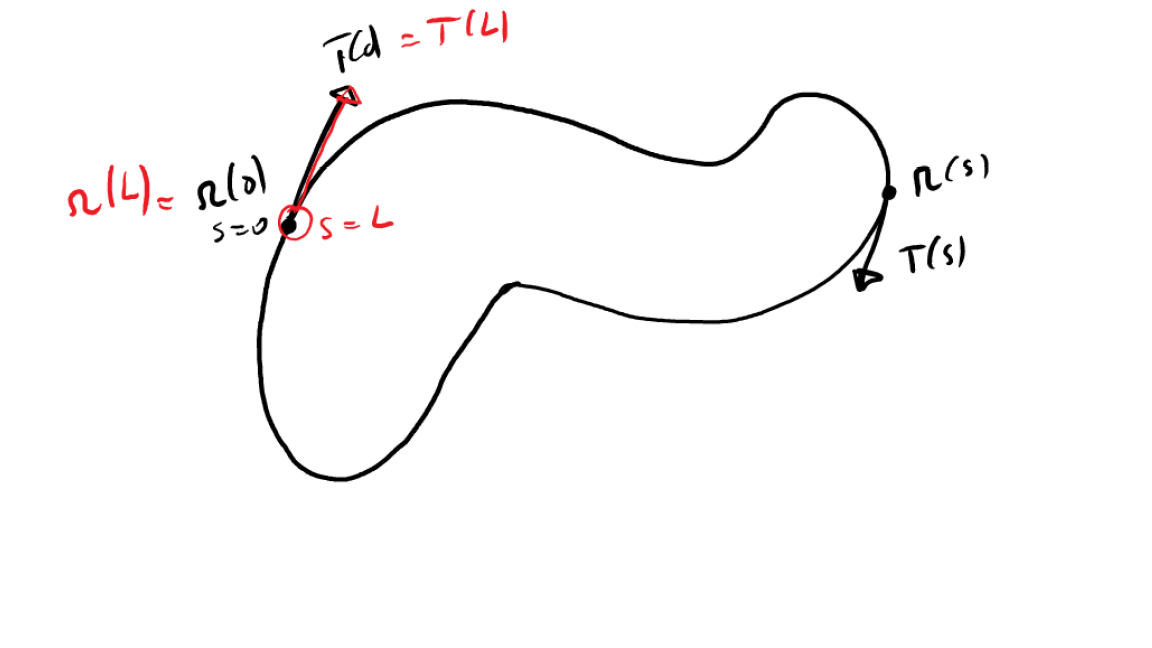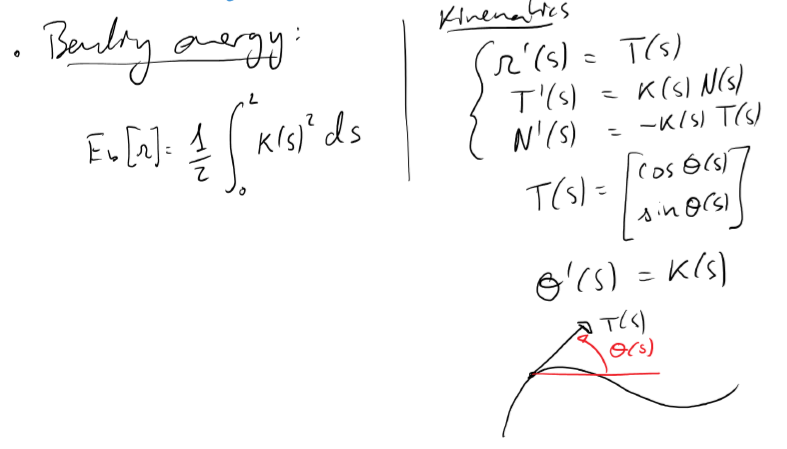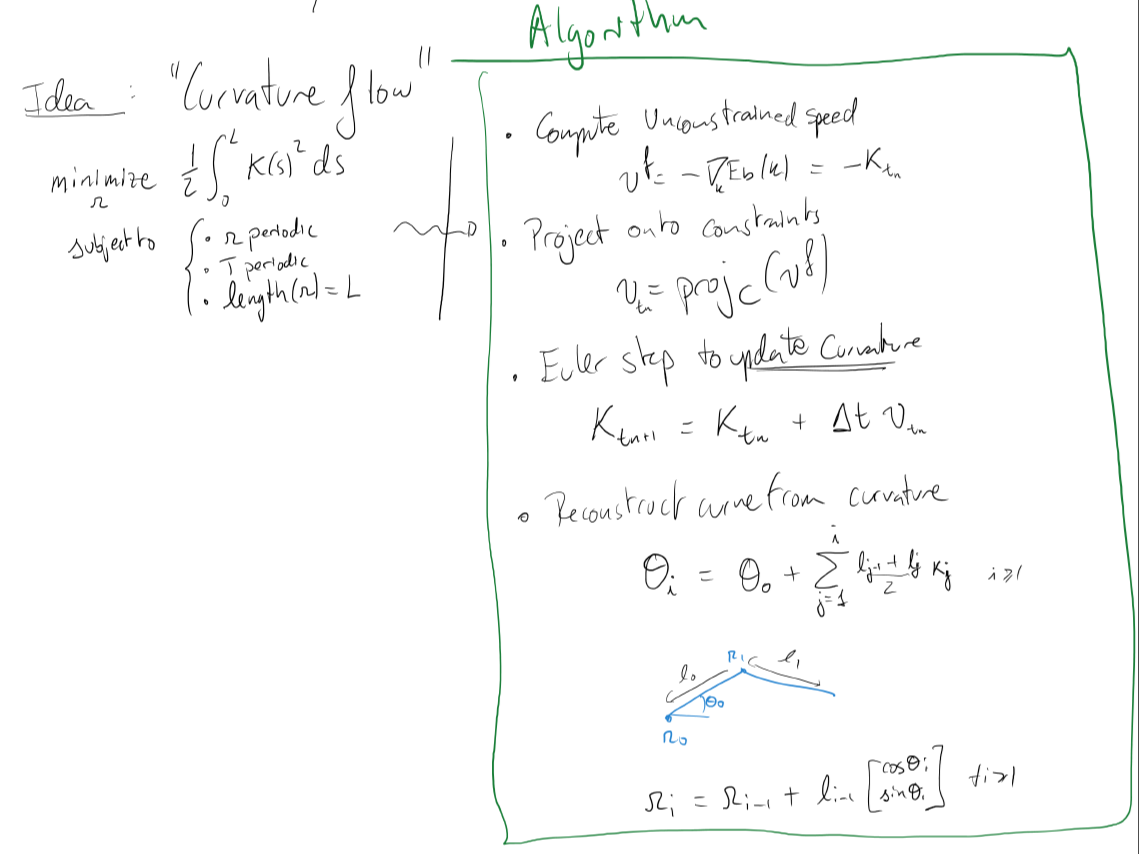Curvature Flow

Using Javascript, p5.js and tf.js to unbend curves

Posted by Alejandro Blumentals on February 9, 2019

I’ve been learning javascript and decided to code up a little curvature flow simulation just for fun. Check it out:

The math behind it is described in this paper by Crane et al. Essentially we’d like to push the vertices of a smooth closed curve in a direction that reduces the total bending energy of the curve while preserving its length and periodicity.Some takeaways

• You can host javascript projects directly on github pages.
• Check out the deployed demo and repo.
• You can directly embed the deployed p5.js sketch in html or jekyll markdown using an iframe.
• You can use vectorized array computations in javascript using tensorflow.js. You don’t have all of the linear algebra functionality of numpy as far as solving linear equations goes. But you do get a Gram Schmidt decomposition, which is just what I needed here.
projectOnConstraints(f) {
// f is an array containing the unconstrained speed
// this function modifies that speed such that the curve remains closed.
let e2 = [];
let e3 = [];
for (let i = 0; i < this.num_points; i++){
e2[i] = this.positions[i].x;
e3[i] = this.positions[i].y;
}
let x1 = tf.ones([this.num_points]);
let x2 = tf.tensor(e2);
let x3 = tf.tensor(e3);
let b = tf.tensor(f);
let A = tf.stack([x1,x2,x3]);
let y = tf.unstack(tf.linalg.gramSchmidt(A));
let res = tf.zerosLike(b);
for(let i = 0; i <3; i++) {
res = tf.add( res, tf.mul(y[i].dot(b),y[i]) )
}
res = tf.sub(b,res);
return res.arraySync();
}